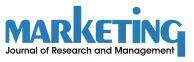# Structural Equation Modeling

(Source: Wikipedia, May 2008)

Structural equation modeling (SEM) is a statistical technique for testing and estimating causal relationships using a combination of statistical data and qualitative causal assumptions. This view of SEM was articulated by the geneticist Sewall Wright (1921), the economists Trygve Haavelmo (1943) and Herbert Simon (1953), and formally defined by Judea Pearl (2000) using a calculus of counterfactuals.

SEM encourages confirmatory rather than exploratory modeling; thus, it is suited to theory testing rather than theory development. It usually starts with a hypothesis, represents it as a model, operationalises the constructs of interest with a measurement instrument, and tests the model. The causal assumptions embedded in the model often have falsifiable implications which can be tested against the data. With an accepted theory or otherwise confirmed model, SEM can also be used inductively by specifying the model and using data to estimate the values of free parameters. Often the initial hypothesis requires adjustment in light of model evidence, but SEM is rarely used purely for exploration.

Among its strengths is the ability to model constructs as latent variables (variables which are not measured directly, but are estimated in the model from measured variables which are assumed to 'tap into' the latent variables). This allows the modeler to explicitly capture the unreliability of measurement in the model, which in theory allows the structural relations between latent variables to be accurately estimated.

In SEM, the qualitative causal assumptions are represented by the missing variables in each equation, as well as vanishing covariances among some error terms. These assumptions are testable in experimental studies and must be confirmed judgmentally in observational studies.

An alternative technique for specifying Structural Models using partial least squares has been implemented in software such as LVPLS (Latent Variable Partial Least Square), PLSGraph and SmartPLS (Ringle et al. 2005). Some feel this is better suited to data exploration. More ambitiously, The TETRAD project aims to develop a way to automate the search for possible causal models from data.

disclaimer

 Published in:German » Sitemap » Disclaimer »